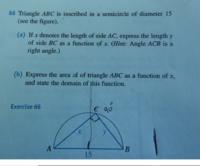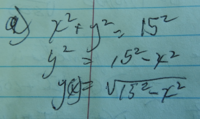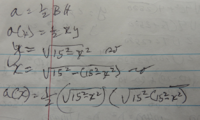# what is the fallacy here?

#### allegansveritatem

##### Full Member
Here is the problem:Here is my solution to part a):Here is my try at first part of part b):This the part of the problem that stumps me. I know there is a fallacy here but...I don't know any other way to proceed. thee must be some reason that the author stresses that the triangle was drawn withing a semicircle. Also I recognize the expression I worked out for part a) describes a semicircle....so how to proceed with this?

#### JeffM

##### Elite Member
Hint. Drop a perpendicular to the diameter from point C, and call the intersection D. So the line CD is the height of the triangle ABC. Now what do we know about triangles ACD and BCD?

•topsquark

#### Dr.Peterson

##### Elite Member
You didn't need to replace x with $$\displaystyle \sqrt{15^2 - (15^2 - x^2)}$$; that's just x!

So your area function is $$\displaystyle A(x) = \frac{1}{2}x\sqrt{225 - x^2}$$. That's the answer. Where do you see a fallacy?

The fact that it's in a semicircle is why you could use the Pythagorean Theorem; you've already used that in part (a), so there's no mystery. All that's left is to state the domain.

#### pka

##### Elite Member
Here is the problem:
View attachment 12525

This the part of the problem that stumps me. I know there is a fallacy here but...I don't know any other way to proceed. thee must be some reason that the author stresses that the triangle was drawn withing a semicircle. Also I recognize the expression I worked out for part a) describes a semicircle....so how to proceed with this?
\displaystyle \begin{align*}x^2+y^2 &=15^2 \\y^2&=15^2-x^2\\y&=\sqrt{15^2-x^2} \end{align*}
If $$\displaystyle \mathscr{A}$$ is the area of $$\displaystyle \Delta ABC$$ then $$\displaystyle \mathscr{A}=\tfrac{1}{2}x\cdot y$$
Thus $$\displaystyle \mathscr{A}=\tfrac{1}{2}x\cdot \sqrt{15^2-x^2}$$

#### allegansveritatem

##### Full Member
You didn't need to replace x with $$\displaystyle \sqrt{15^2 - (15^2 - x^2)}$$; that's just x!

So your area function is $$\displaystyle A(x) = \frac{1}{2}x\sqrt{225 - x^2}$$. That's the answer. Where do you see a fallacy?

The fact that it's in a semicircle is why you could use the Pythagorean Theorem; you've already used that in part (a), so there's no mystery. All that's left is to state the domain.
Well, the reason I used the crazy substitution for x is...I guess I thought that since y=sqrt 15^2-x^2 then x would be sqrt15^2-y^2 or sqrt15^2-x^2. I still haven't absorbed your psot so I don't know exactly the why of it. I will work with it later in the day. As for using the Pythagorean theorem, wouldn't that have been assured by telling us that the triangle is a right triangle? Why would we need to know that the figure had been inscribed in a semicircle?

#### allegansveritatem

##### Full Member
\displaystyle \begin{align*}x^2+y^2 &=15^2 \\y^2&=15^2-x^2\\y&=\sqrt{15^2-x^2} \end{align*}
If $$\displaystyle \mathscr{A}$$ is the area of $$\displaystyle \Delta ABC$$ then $$\displaystyle \mathscr{A}=\tfrac{1}{2}x\cdot y$$
Thus $$\displaystyle \mathscr{A}=\tfrac{1}{2}x\cdot \sqrt{15^2-x^2}$$
I have to absorb this and explain to myself why it is so., which I will do later this morning. I don't doubt you are right but I have to "do the math" to see it. Thanks

#### Dr.Peterson

##### Elite Member
Well, the reason I used the crazy substitution for x is...I guess I thought that since y=sqrt 15^2-x^2 then x would be sqrt15^2-y^2 or sqrt15^2-x^2. I still haven't absorbed your psot so I don't know exactly the why of it. I will work with it later in the day. As for using the Pythagorean theorem, wouldn't that have been assured by telling us that the triangle is a right triangle? Why would we need to know that the figure had been inscribed in a semicircle?
The semicircle is WHY you can know it's a right triangle. The problem itself only stated the former; the HINT represents what you have to deduce from that. It shouldn't have been given, in my opinion, because it makes the problem too easy by not making you realize that yourself.

I imagine the reason you did the unnecessary substitution was mere momentum! You did that for y, so you did it for x as well. It wasn't wrong; it just took you past your goal so you had to stop, think, and back up. (Yes, I'm picturing the "momentum" literally.)

#### allegansveritatem

##### Full Member
Yes, momentum. Another way of looking at it (or is it the same?) is I was primed by the process of finding the first substitution to continue in the same mode. Anyway, after reading yours and pka's posts I went back at it today and the scales fell away from my eyes. I was gilding the lily. I already had a perfectly god X and here I was cobbling up another. Where was Occam when I needed him?
Re: the semicircle. I'm afraid I am not up on the geometry of semicircles and lines enough to be able to deduce that lines drawn from opposite ends of the diameter of a semicircle that meet on the circumference always form a right triangle with the diameter being the hypotenuse. I have in fact just now deduced this after reading you post and going back to the illustration. Is that what you mean when you say that "the semicircle is WHY you can know it's a right triangle?

#### pka

##### Elite Member
Is that what you mean when you say that "the semicircle is WHY you can know it's a right triangle?
To understand an answer one needs to know that an inscribed angle is one with its vertex in the circle and then the arc of the circle between the two points of the angle that are on the circle is called the intercepted arc. For provable reasons the measure of an inscribed angle is one-half the measure of the intercepted are. The length of the circle is $$\displaystyle 2\pi\cdot R$$ where $$\displaystyle R$$ is the radius of the circle. We drop the $$\displaystyle R$$ and say simply the the measure of the entire circle is $$\displaystyle 2\pi$$. One-half that is simply $$\displaystyle \pi$$ that the measure of a semi-circle. This an inscribed angle in a semi-circle must intercept a semi-circle hence its measure is $$\displaystyle \frac{\pi}{2}$$ which is a right angle.